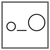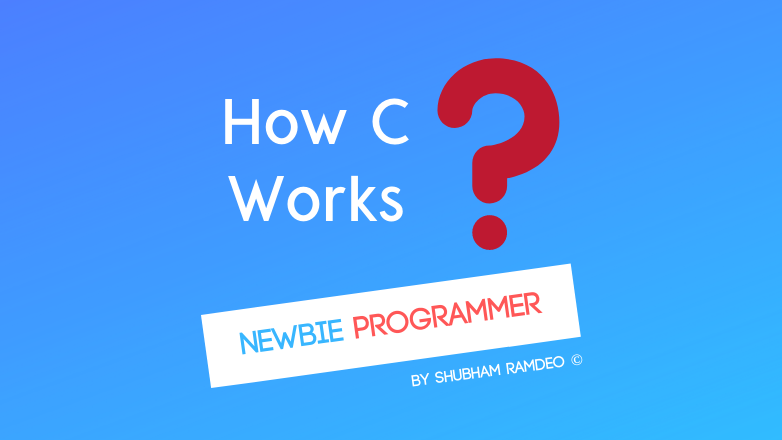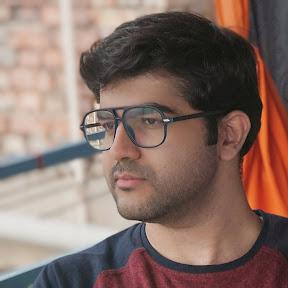Fuddlepixel coz tech is fun();

# Scope of the variables Inside outside of a function

Let us discuss how the scope of variables can be set to make them work according to our needs inside or outside of a function in the C language.

By Shubham Ramdeo
14 October 2015 · 4 mins read
# `c`  `newbie`That’s how the scope of the variables can be set in and out of a function !

Welcome to the Newbie Programmer Series. We were doing functions till the last part. We have learned how to use functions, their different ways of declarations, and also some tricks like recursion ( that’s hard, we will do it again ).  In this part, we will discuss the concept called Scope. We will learn using variables in different ways in and out of the functions. So if you are new to this series, please go to index ( click here ) and read out all the previous parts so that you can easily understand what I am writing about.

By the way, I apologies for not posting any article since a week. But that’s not my fault. There is some construction going on and that has cut down the internet cables of my house. So I was unable to connect through the internet.

This chapter is divided into two parts. What you are reading now is part 1. We will do part 2 later. Also I am doing large articles that I should not do as it become too much and harder to understand so from now I will try to divide large ones into small parts.

Lets get started. Oh, I mean,

I might not have written it clearly but We can declare as many functions as we want and wherever we want.

For example :

``````#include <stdio.h>
say_hello()
{
printf("Hello ! \n");
}

say_bye()
{
printf("Bye ! \n");
}

main()
{
say_hello();
say_bye();
}
``````

OUTPUT:

``````Hello !
Bye !
``````

Here we have made two functions and used both of them in the main function.

NOTE : But We cannot make functions inside functions as we do with loops inside loops.

The concept of Scope come into play when we make many functions with many variables. Scope means up to what limits a variable can be used.

Lets discuss the basic. We can define variables in two ways.

1. Define variables inside a function. These variables can be used inside a function only and will destroy once the function is complete.
2. Define variables outside. These variables can be used by any function and will destroy only when the program itself ends.

For an example :

``````#include<stdio.h>
int pi = 3.14;
main()
{
printf("The circumference of that circle is %d \n", circum( radius ) );
printf("The area of that circle is %d \n", area( radius ) );
return 0;
}

int r;

int area ( r )
{
int ar = pi * r * r;
return ar;
}

int circum ( r )
{
int c = 2 * pi * r;
return c;
}
``````

In the above we have the variables “pi” and “r” outside any function. So they can be used by any function. We have declared “float pi = 3.14;” and then we used it inside area and circum function without declaring it. We also did the same with the variable “r”. You see I have NOT DECLARED variable r in circum and area functions.

But the variables which are declared inside some functions like “radius”, “a”, “c” cannot be used by other functions. These variables can be used by that function only in which they are declared.

So you add this line in the main function :

``````printf("Value of pi is %f \n", pi );
``````

will successfully print “Value of pi is 3.14”.

This is because pi variable is defined outside any function body so it can be used by all the functions in the program.

``````printf("You entered radius %f \n", radius );
``````

Will also successfully print the number you have entered.

This is because the radius variable is defined inside the main function so it can be used by main. BUT NO OTHER FUNCTION.

``````printf(" the value of a is %f", a );
``````

OR

``````printf(" the value of c is %f", c );
``````

OR

``````printf(" the value of test is %f", test );
``````

Will cause error as main function do not know what is variable “a”, “c” or “test” is. These variables are used in area and circum functions and can be used by those respective functions only and no other.

Now that was the first part of the scope that tells us how the variables inside or outside a function body can be used in different ways. In the next part of the scope, we will extern and static and register variables. But before that, We should know how we can use many files for a single program and also about using header files. So first we will do that and will discuss scope part two after that.

So as part 1 ended and I have nothing to write more about this part 1 topic, I think we did enough for today, you may play some more. We are gonna discuss more interesting stuffs in the next part. So stay connected.

##### Update me weekly ✉

Subscribe below and we’ll send you a weekly email summary of all new Code tutorials. Never miss out on learning about the next big thing.

#### Shubham RamdeoShubham Ramdeo is the founder of Fuddlepixel. He is a geek, a writer, involved with many technical and humanity projects.

Meet me!

#### Recent#### Related

`c`

`newbie`

Top